Note

This page is a reference documentation. It only explains the function signature, and not how to use it. Please refer to the user guide for the big picture.

# nilearn.plotting.plot_design_matrix#

nilearn.plotting.plot_design_matrix(design_matrix, rescale=True, ax=None, output_file=None)[source]#

Plot a design matrix provided as a `pandas.DataFrame`.

Parameters:
design matrix`pandas.DataFrame`

Describes a design matrix.

rescale`bool`, optional

Rescale columns magnitude for visualization or not. Default=True.

ax`matplotlib.axes.Axes`, optional

Handle to axes onto which we will draw the design matrix.

output_file`str`, or None, optional

The name of an image file to export the plot to. Valid extensions are .png, .pdf, .svg. If output_file is not None, the plot is saved to a file, and the display is closed.

Returns:
ax`matplotlib.axes.Axes`

The axes used for plotting.

## Examples using `nilearn.plotting.plot_design_matrix`#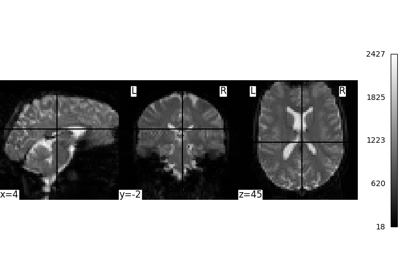Intro to GLM Analysis: a single-session, single-subject fMRI dataset

Intro to GLM Analysis: a single-session, single-subject fMRI dataset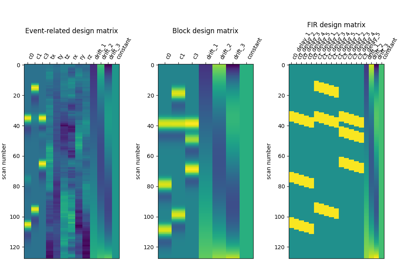Examples of design matrices

Examples of design matrices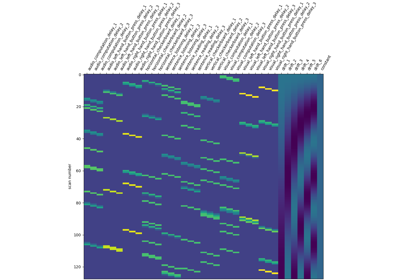Analysis of an fMRI dataset with a Finite Impule Response (FIR) model

Analysis of an fMRI dataset with a Finite Impule Response (FIR) model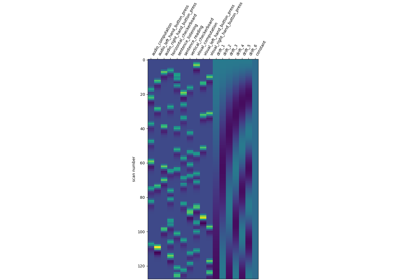Understanding parameters of the first-level model

Understanding parameters of the first-level model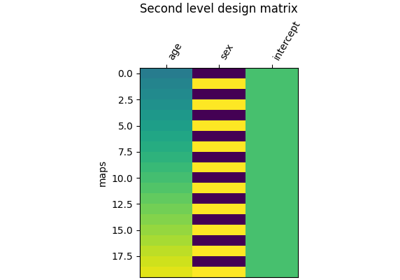Example of second level design matrix

Example of second level design matrix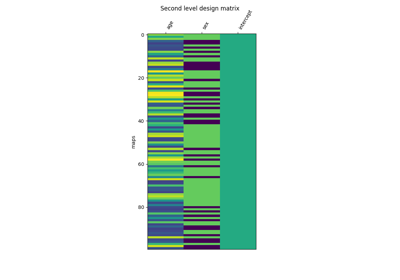Voxel-Based Morphometry on OASIS dataset

Voxel-Based Morphometry on OASIS dataset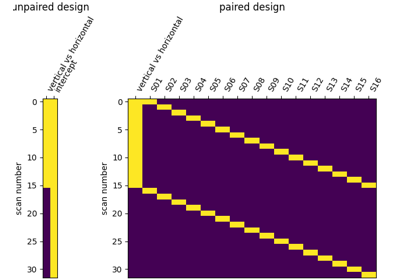Second-level fMRI model: two-sample test, unpaired and paired

Second-level fMRI model: two-sample test, unpaired and paired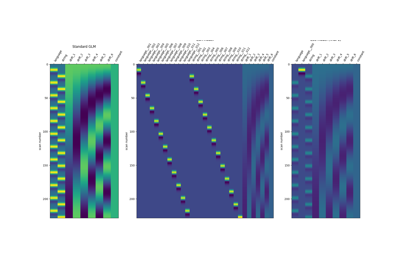Beta-Series Modeling for Task-Based Functional Connectivity and Decoding

Beta-Series Modeling for Task-Based Functional Connectivity and Decoding